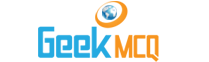Entry Test MCQ :: Work And EnergyAn elevator weighing 3.5 x 10-6 N is raised to a height of 1000 m in the absence of friction, the work done is
 [A]. 3.5 x 103 J [B]. 3.5 x 104 J [C]. 3.5 x 106 J [D]. 3.5 x 109 Jsalman said: Why D option is correct give me prove

 sahar said: d is not the answer.the correct answer is 3.5x10^-3J which is not present in given options.

 ali shah said: 3.5 exp -3 is the perfect answer ... correct it asap

 parkash umedani said: o said option is correct

 M.Bilal majeed said: d is not the answer.the correct answer is 3.5x10^-3J which is not present in given options.

 AbdulMoiz said: Plz tell me which formula is used here?

 azmat said: formula used is W=F.d 3.5x10N-6X1000m=3.5x10-3j

 Shabana said: yes ofcourse this option d is wrong correct is 3.5x10-3j

 shabana said: yes ofcourse the correct ans is 3.5x10-3j

 shanshah said: ji correct 3.5x10-3j q ke w=p.e= weightxheight